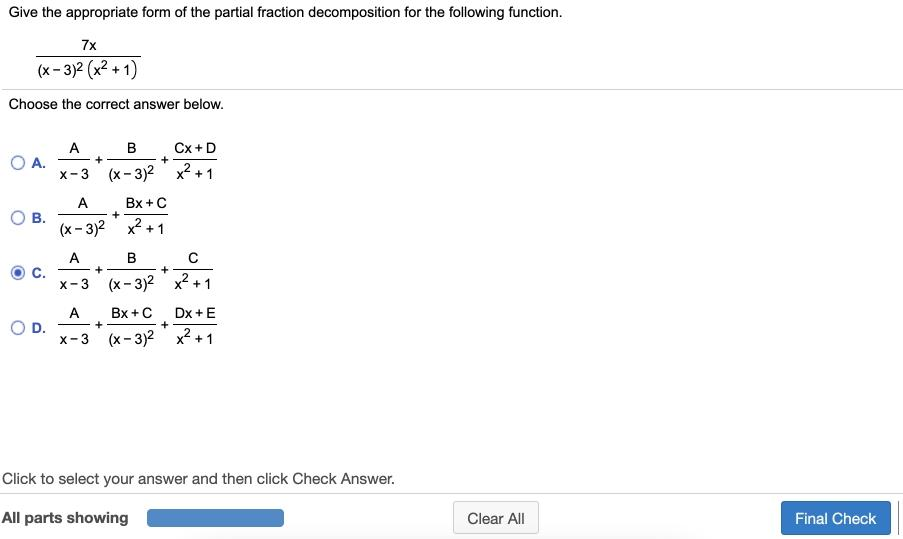# Question Give the appropriate form of the partial fraction decomposition for the following function. Give the appropriate form of the partial fraction decomposition for the following function. 7x (x - 3)2(x2 + 1) Choose the correct answer below. OA. + + ОВ. + A B Cx+D X-3 (x - 3)2 x2 +1 A Bx + C (x - 3)2 x2 +1 A B с X-3 (x - 3)2 x2 +1 A BX+C Dx+E x-3 (x-3) 2x2+1 + + C. + + OD. Click to select your answer and then click Check Answer. All parts showing Clear All Final Check